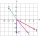# Linear independence

Determine if vectors u=(-4; -5) and v=(20; 25) are linear Linear dependent.

Result

#### Solution:

$k = 20/(-4) = 25/(-5) = -5 \ \\ v = -5 u$

Leave us a comment of this math problem and its solution (i.e. if it is still somewhat unclear...):Be the first to comment!#### Following knowledge from mathematics are needed to solve this word math problem:

For Basic calculations in analytic geometry is helpful line slope calculator. From coordinates of two points in the plane it calculate slope, normal and parametric line equation(s), slope, directional angle, direction vector, the length of segment, intersections the coordinate axes etc. Two vectors given by its magnitudes and by included angle can be added by our vector sum calculator. Do you have a linear equation or system of equations and looking for its solution? Or do you have quadratic equation?

## Next similar math problems:

1. CuboidsTwo separate cuboids with different orientation in space. Determine the angle between them, knowing the direction cosine matrix for each separate cuboid. u1=(0.62955056, 0.094432584, 0.77119944) u2=(0.14484653, 0.9208101, 0.36211633)
2. Unit vector 2DDetermine coordinates of unit vector to vector AB if A[-6; 8], B[-18; 10].
3. Find the 5Find the equation with center at (1,20) which touches the line 8x+5y-19=0
4. Three points 2The three points A(3, 8), B(6, 2) and C(10, 2). The point D is such that the line DA is perpendicular to AB and DC is parallel to AB. Calculate the coordinates of D.
5. Find the 10Find the value of t if 2tx+5y-6=0 and 5x-4y+8=0 are perpendicular, parallel, what angle does each of the lines make with the x-axis, find the angle between the lines?
6. LineLine p passing through A[-10, 6] and has direction vector v=(3, 2). Is point B[7, 30] on the line p?
7. Angle between vectorsFind the angle between the given vectors to the nearest tenth of a degree. u = (-22, 11) and v = (16, 20)
8. Scalar productCalculate the scalar product of two vectors: (2.5) (-1, -4)
9. Vector sumThe magnitude of the vector u is 12 and the magnitude of the vector v is 8. Angle between vectors is 61°. What is the magnitude of the vector u + v?
10. VectorsVector a has coordinates (8; 10) and vector b has coordinates (0; 17). If the vector c = b - a, what is the magnitude of the vector c?
11. TriangleTriangle KLM is given by plane coordinates of vertices: K[11, -10] L[10, 12] M[1, 3]. Calculate its area and itsinterior angles.
12. Coordinates of square verticesThe ABCD square has the center S [−3, −2] and the vertex A [1, −3]. Find the coordinates of the other vertices of the square.
13. SquarePoints A[-9,7] and B[-4,-5] are adjacent vertices of the square ABCD. Calculate the area of the square ABCD.The rectangular crossroads comes passenger car and an ambulance, the ambulance left. Passenger car is at 36 km/h and ambulance 47 km/h. Calculate such a relative speed of the ambulance moves to the car.Two forces with magnitudes of 25 and 30 pounds act on an object at angles of 10° and 100° respectively. Find the direction and magnitude of the resultant force. Round to two decimal places in all intermediate steps and in your final answer.An airplane leaves an airport and flies to west 120 miles and then 150 miles in the direction S 44.1°W. How far is the plane from the airport (round to the nearest mile)?Calculate length of the vector v⃗ = (9.75, 6.75, -6.5, -3.75, 2).